Geometric Shapes

## Geometric Shapes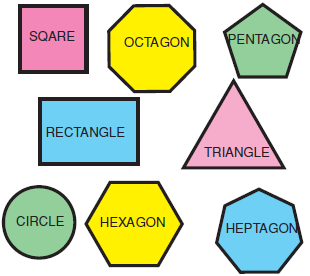Activity Exercise

Find the name of the shapes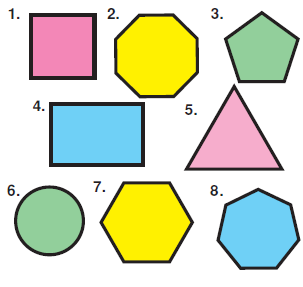1. square 2. octagon 3. pentagon 4. rectangle
5. triangle 6. circle 7. hexagon 8. heptagon

How many squares are there in the picture?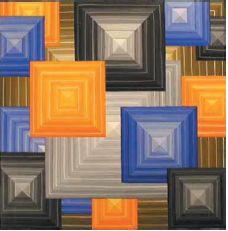fourteen squares

Match with the pictures
1.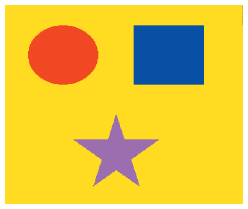2.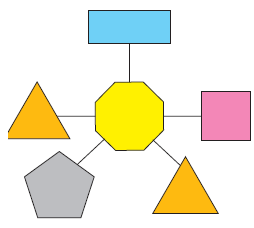3.4.a) There is a square, a circle and a star,
b) There are two triangles and three circles.
c) There are two triangles, an octagon, a pentagon, a square and a rectangle.
d) There are eight triangles six ellipses, seven circles, three squares and four rectrangles.

1. a 2. c 3. d 4. b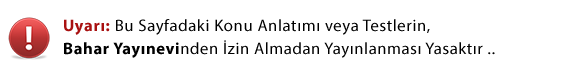Sınavlara Hazırlık Arama Robotu
YGS & LYS TEOG KPSS TUS KPDS Ehliyet Sınavı PMYO JANA

Seçim esnek olup ilgili alanları seçiniz, Örneğin ehliyet sınavı için branş olarak matematik seçmeyiniz :)